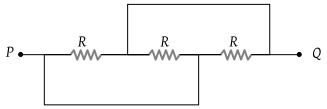# NEET Questions Solved

PMT - 2002

The equivalent resistance between the points P and Q of the circuit given is(1) $\frac{R}{4}$

(2) $\frac{R}{3}$

(3) 4 R

(4) 2 R

(2) All of three resistance are in parallel So, $R\text{'}=R\text{​}/\text{​}n=\frac{R}{3}$

Difficulty Level:

• 8%
• 74%
• 15%
• 5%